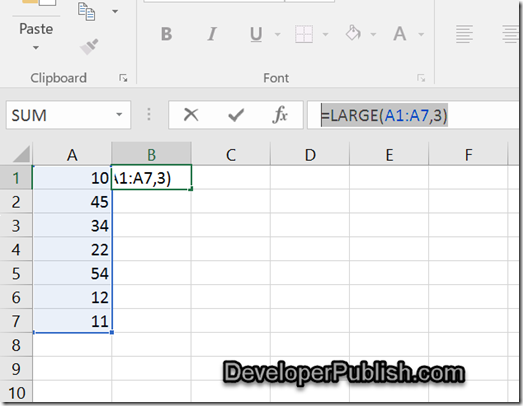How to find the Nth Largest Numbers from a List of values in Excel ? - Developer Publish

# How to find the Nth Largest Numbers from a List of values in Excel ?

There are times when you might want to find out the 3rd Largest value from the list of number from Excel cells.

# How to find the N Largest Numbers from a List of values in Excel ?

Microsoft Excel provides the function LARGE which lets you to this.

The function LARGE takes 2 parameters.
– The first parameter takes the range of cells.
– The second parameter specifies the nth Largest value that you are searching for.

Assume that the Excel Column (A1:A7) contains the range of values , you would need to find the 3rd Largest value from this range and display it in the cell B1.

Just enter the following formula in the cell B1.

=LARGE(A1:A7,3)